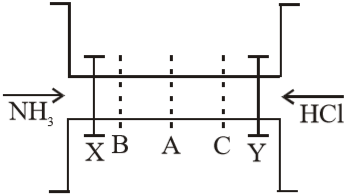The valves X and Y are opened simultaneously. The white fumes of ${\mathrm{NH}}_{4}\mathrm{Cl}$ will first form at :(A) A

(B) B

(C) C

(D) A, B and C simultaneously

Concept Videos :-

#6 | Graham's Law of Diffusion

Concept Questions :-

Graham's Law

(C).  Since rate of diffusion of ${\mathrm{NH}}_{3}$ is more then $\mathrm{HCl}$ due to its comparatively lower mol. wt.,

therefore, fumes of ${\mathrm{NH}}_{4}\mathrm{Cl}$ will be formed at C.

Difficulty Level:

• 6%
• 17%
• 58%
• 21%